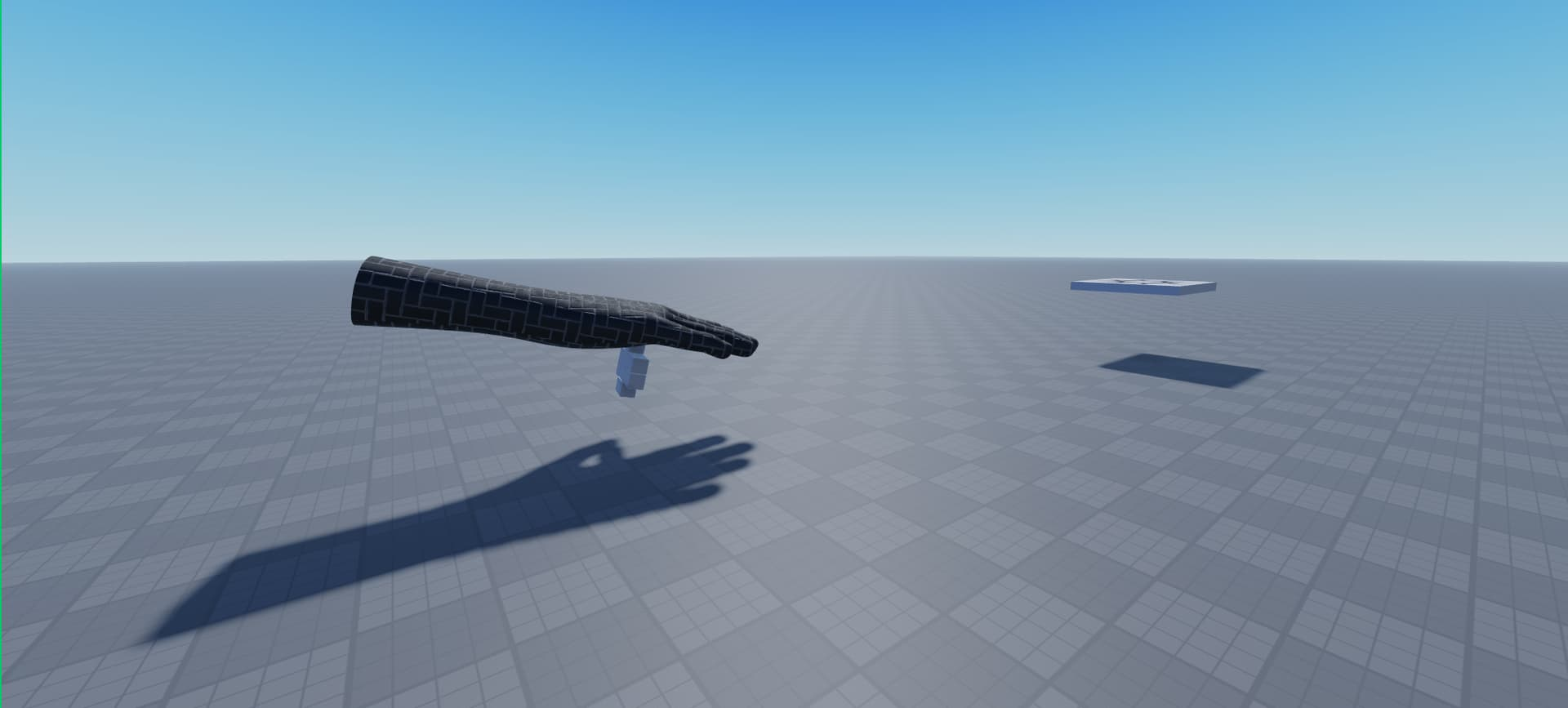# How do I make part look at part?

I want the hand to look at the part, but with edited orientation. I want the hand rotated like a twisting way.

``````script.Parent["Cube.000"].CFrame = CFrame.lookAt(script.Parent["Cube.000"].Position,workspace.SpawnLocation.Position) * CFrame.Angles(math.rad(0),math.rad(-90),math.rad(15))
``````

The CFrame.Angles, barely works, its messsed up. X, rotates the hand up. Y, rotates the hand left/right.Z, rotates the hand down??? Why isn’t there a third one? Why does x and z basically do the same thing??? (sorry for the awkward hand pose)I want to rotate it like this

nvm i fixed it `* CFrame.Angles(math.rad(-90),math.rad(0),math.rad(-90))`